Test: Coulomb's Law

# Test: Coulomb's Law

Test Description

## 10 Questions MCQ Test Electromagnetic Fields Theory (EMFT) | Test: Coulomb's Law

Test: Coulomb's Law for Electrical Engineering (EE) 2023 is part of Electromagnetic Fields Theory (EMFT) preparation. The Test: Coulomb's Law questions and answers have been prepared according to the Electrical Engineering (EE) exam syllabus.The Test: Coulomb's Law MCQs are made for Electrical Engineering (EE) 2023 Exam. Find important definitions, questions, notes, meanings, examples, exercises, MCQs and online tests for Test: Coulomb's Law below.
Solutions of Test: Coulomb's Law questions in English are available as part of our Electromagnetic Fields Theory (EMFT) for Electrical Engineering (EE) & Test: Coulomb's Law solutions in Hindi for Electromagnetic Fields Theory (EMFT) course. Download more important topics, notes, lectures and mock test series for Electrical Engineering (EE) Exam by signing up for free. Attempt Test: Coulomb's Law | 10 questions in 30 minutes | Mock test for Electrical Engineering (EE) preparation | Free important questions MCQ to study Electromagnetic Fields Theory (EMFT) for Electrical Engineering (EE) Exam | Download free PDF with solutions
 1 Crore+ students have signed up on EduRev. Have you?
Test: Coulomb's Law - Question 1

### If two same charges are 3 x 10-7 C and acted upon by a force of 0.5N. Determine the distance between them, if both the charges are in a vacuum.

Detailed Solution for Test: Coulomb's Law - Question 1

Concept:
According to Coulomb's law, the electrostatic force between two point charges is directly proportional to the product of their magnitudes and inversely proportional to the square of the distance between their centres.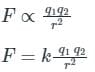where,
F = Electrostatic force
q1 and q2 are point charges
r = distance between two point charge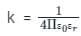= Proportionality constant
where,
ε0 = Absolute permittivity of vacuum or air
εr = Relative permittivity of the medium in which charges are placed. For vacuum or air, its value is 1
The value of ε0 = 8.854 × 10-12 F/m
Calculation:
Given,
q1 = q2 = 3 x 10-7 C
F = 0.5N
∵ The medium is vacuum so εr = 1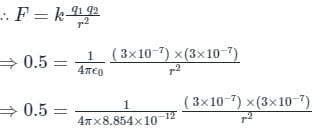⇒ r = 0.04 m

Test: Coulomb's Law - Question 2

### The constant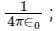represents which of the following?

Detailed Solution for Test: Coulomb's Law - Question 2

It states that the force of interaction between two stationary point charges is directly proportional to the product of the charges, and inversely proportional to the square of the distance between them and acts along the straight line joining the two charges.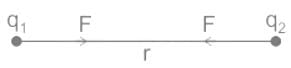F ∝ q1 ∝ q2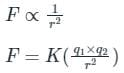Where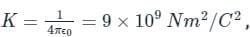also called Coulomb's constant or electrostatic force constant.
Here ϵ0 = Permittivity of free space.
The value of K depends on the nature of medium between the two charges and the system of units chosen.

Test: Coulomb's Law - Question 3

### The electric field of a point charge is a linear function of the value of the charge. It is the principle applied to an electric field and states that the total resultant field at a point is the vector sum of the individual components of the field at a point. Identify the principle mentioned in the context.

Detailed Solution for Test: Coulomb's Law - Question 3

CONCEPT:
Superposition Principle:

• The principle of superposition states that when a number of charges are interacting, the total force on a given charge is the vector sum of the forces exerted on it due to all other charges.
• The force between two charges is not affected by the presence of other charges.
• This is used to calculate the following parameter at the observation point, to any configuration of charges.
• Net force.
• Net electric field.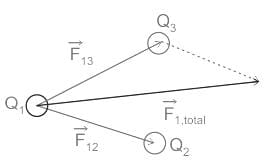Coulomb's law in Electrostatics:

• It state’s that force of interaction between two stationary point charges is directly proportional to the product of the charges, and inversely proportional to the square of the distance between them and acts along the straight line joining the two charges.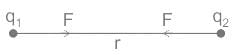F ∝ q1 × q2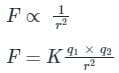Where K = constant called electrostatic force constant.

• The value of K depends on the nature of the medium between the two charges and the system of units chosen
Test: Coulomb's Law - Question 4

Two charges of + 4 μC and -16 μC are separated from each other by a distance of 0.6 m. At what distance should a third charge of + 6 μC be placed from + 4 μC so that net force exerts on it will be zero?

Detailed Solution for Test: Coulomb's Law - Question 4

CONCEPT:
Coulomb’s law: When two charged particles of charges q1 and q2 are separated by a distance r from each other then the electrostatic force between them is directly proportional to the multiplication of charges of two particles and inversely proportional to the square of the distance between them.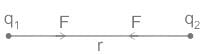Force (F) ∝ q1 × q2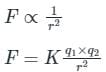Where K is a constant = 9 × 109 Nm2/C2
Calculations:
Consider new charge + 4μC is placed d m apart from old +4 μC charge and (x + 0.6) m apart from -16 μC charge.

Let,
qA = + 4 μC at point A
qB = - 16 μC at point B
qC = + 6 μC at point C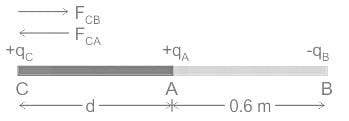Since, net force on charge qC will zero.
∴ |FCA| = |FCB|
From above concept,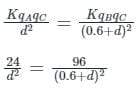4d2 = (0.6 + d)2
4d2 = 0.36 + d2 + 1.2d
3d2 - 1.2d - 0.36 = 0
d1 = - 0.2 m
d2 = + 0.6 m
Hence, according to option + 0.6 m distance should a third charge of + 6 μC be placed from + 4 μC so that no force exerts on it will be zero.

Test: Coulomb's Law - Question 5

The force between two charges Q1 and Q2 is given by :

Detailed Solution for Test: Coulomb's Law - Question 5

Coulomb’s Law: It states that the force between two charged particles will be directly proportional to the product of the quantity of the two charges.
F ∝ Q1Q2,
Secondly, it is also inversely proportional to the square of the distance between them, i.e.
F ∝ 1/r2
∴ The force between two charges Q1 and Q2 is given by: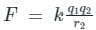where k is the proportionality constant and is equal to 9 × 109 Nm2/C2.

Test: Coulomb's Law - Question 6

Consider the two point particles separated by a distance 'd' have charges Q1 and Q2 respectively. Particle Q2 experiences an electrostatic force of 20 mN due to particle Q1. If the charges of both particles are doubled and distance between them is also doubled, what is the magnitude of the electrostatic force between them?

Detailed Solution for Test: Coulomb's Law - Question 6

Concept:

• Coulomb’s law: When two charge particles of charges q1 and q2 are separated by a distance r from each other then the electrostatic force between them is directly proportional to multiplication of charges of two particles and inversely proportional to square of distance between them.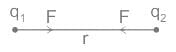Force (F) ∝ q1 × q2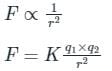Where K is a constant = 9 × 109 Nm2/C2
Case 1:
Given that charges are: Q1 and Q00
Distance = d
Force (F1) = 20 mN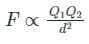Case 2:
The charges of both particles are doubled and distance between them is also doubled.
Given that charges are: 2Q1 and 2Q2
Distance = 2d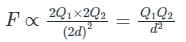Therefore, the force remains same and it is equal to 20 mN

Test: Coulomb's Law - Question 7

False statement among the following statements regarding Coulombs law is:

Detailed Solution for Test: Coulomb's Law - Question 7

CONCEPT:
Coulomb’s law: When two charged particles of charges q1 and q2 are separated by a distance r from each other then the electrostatic force between them is directly proportional to the multiplication of charges of two particles and inversely proportional to the square of the distance between them.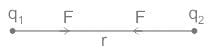Force (F) ∝ q1 × q2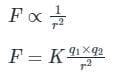Where K is a constant = 9 × 109 Nm2/C2

• Coulomb’s Law talks about the magnitude of the attraction between the two charges.
• It says that the force is directly proportional to the product of the quantity of the two charges and inversely proportional to the square of the distance between them.
Test: Coulomb's Law - Question 8

According to which law, the direction of induced emf in the choke of a tube light will be such that it will try to oppose the fall of the current in the circuit?

Detailed Solution for Test: Coulomb's Law - Question 8

Lenz’s Law:

• It states that the direction of the current induced in a conductor by a changing magnetic field is such that the magnetic field created by the induced current opposes the initial changing magnetic field.
• When a current is induced by a magnetic field, then the magnetic field produced by the induced current will create its magnetic field.
• Thus, this magnetic field will be opposed by the magnetic field that created it.
Test: Coulomb's Law - Question 9

A charge Q2 = 8.854 × 10-9 C is located in vacuum at P2 (2, 3, 1). The force on Q2 due to a charge Q1 = 4π × 10-3 C at P1 (2, 2, 1) is:
(Note: All the coordinates are measured in meters ax, ay, and az are unit vectors in X, Y, and Z direction respectively.)

Detailed Solution for Test: Coulomb's Law - Question 9

Concept:
The force acting on a point charge because of another point charge is given as: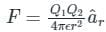(Where âr is a unit vector in the direction of the force)
Calculation:
Given Q1 = 4π × 10-3 C
Q2 = 8.854 × 10-9 C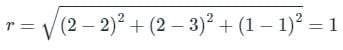Since, we are asked to find the force on Q2 due to Q1, the direction of the force
= âr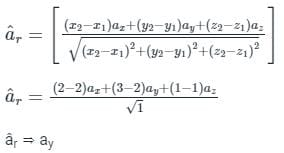So, the Force on Q2 due to Q1 will be: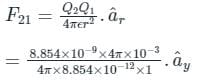= ay N

Test: Coulomb's Law - Question 10

The law, governing the force between electric charges is known as:

Detailed Solution for Test: Coulomb's Law - Question 10

CONCEPT:
Coulomb’s law: When two charged particles of charges q1 and q2 are separated by a distance r from each other then the electrostatic force between them is directly proportional to the multiplication of charges of two particles and inversely proportional to the square of the distance between them.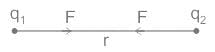Force (F) ∝ q1 × q2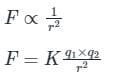Where K is a constant = 9 × 109 Nm2/C2

• Coulomb’s Law talks about the magnitude of the attraction between the two charges.
• It says that the force is directly proportional to the product of the quantity of the two charges and inversely proportional to the square of the distance between them.

## Electromagnetic Fields Theory (EMFT)

11 videos|46 docs|62 tests
 Use Code STAYHOME200 and get INR 200 additional OFF Use Coupon Code
Information about Test: Coulomb's Law Page
In this test you can find the Exam questions for Test: Coulomb's Law solved & explained in the simplest way possible. Besides giving Questions and answers for Test: Coulomb's Law, EduRev gives you an ample number of Online tests for practice

## Electromagnetic Fields Theory (EMFT)

11 videos|46 docs|62 tests